How to Add Brackets for Cells in Excel

Sometimes we need to add brackets into cells to enclose texts for some reasons. If we only add brackets for one single cell, it is very easy, but if there are a column of cells need to add brackets, how can we do? This article will show you three simple ways to add brackets by Formula, Format Cells Function and VBA code.

In condition, we prepare a table to save Year.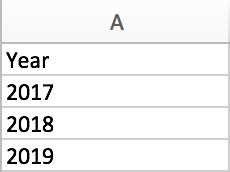We use this sample to do demonstration.

Add Brackets for Cells by Formula in Excel

Step 1: Select another column for example column B, in cell B2 enter =”(“&A2&”)”.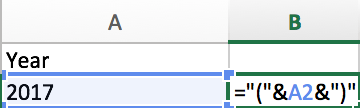Step 2: Press Enter to get result.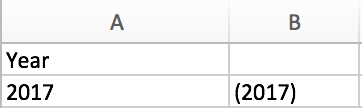Step 3: Select cell B2 which contains the formula, drag it and fill the cells B3 and B4. Then we can get a new column with brackets enclose the texts. You can copy the result to cover column A by paste with value.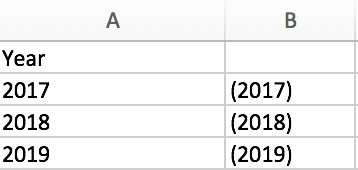Add Brackets for Cells by Format Cells Function in Excel

Step 1: Select all ranges you want to add brackets for them. In this case select A2, A3 and A4.

Step 2: Right click your mouse, select ‘Format Cells…’.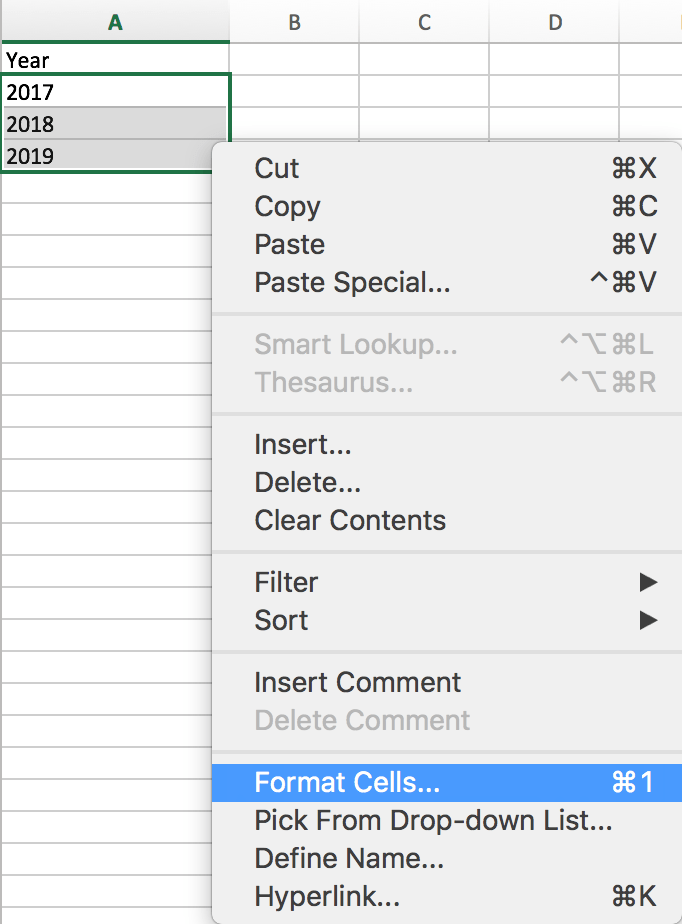Step 3: On Format Cells window, select Custom under Category list.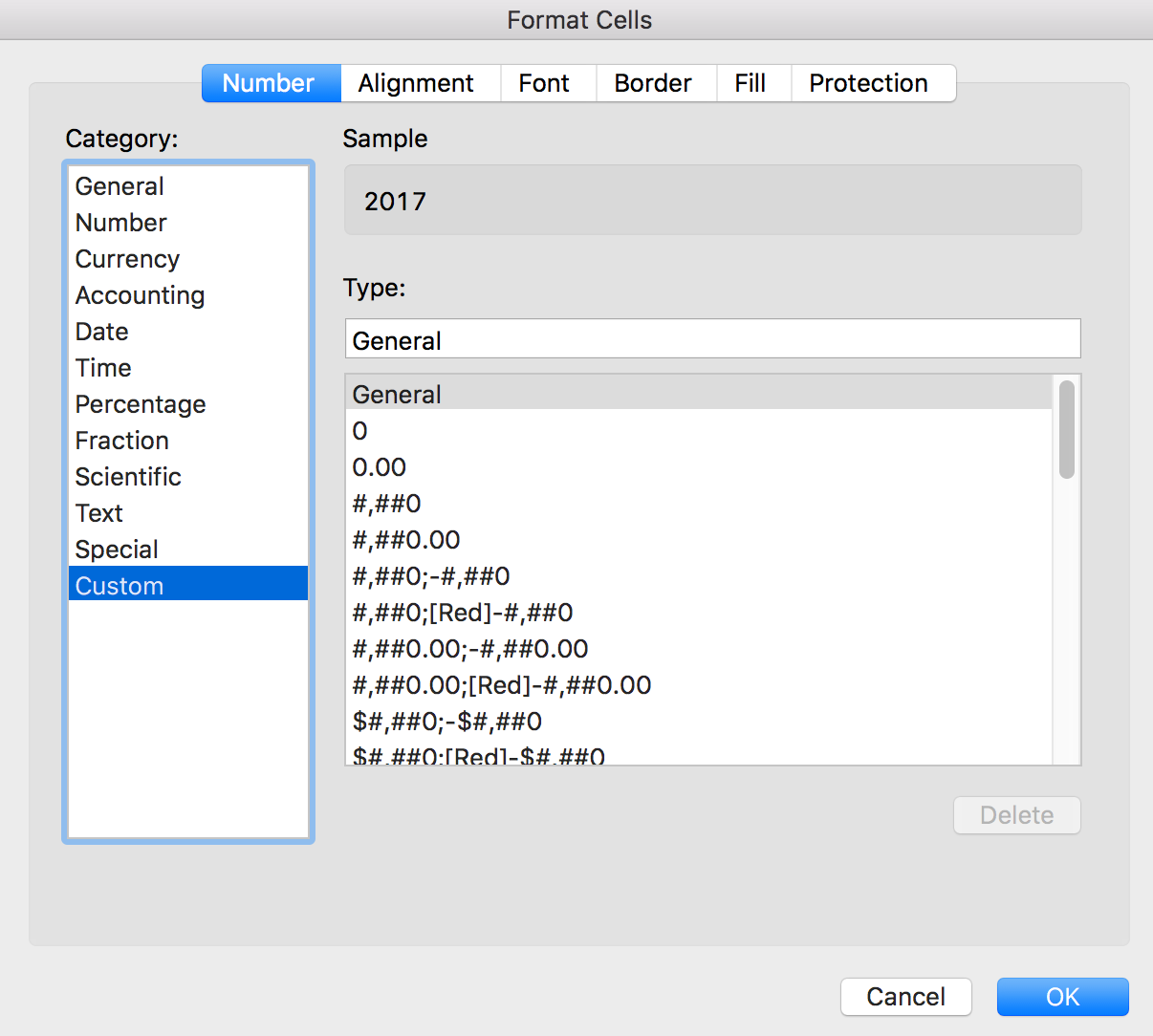Step 4: In Type, enter “(“General”)”. Then you can find 2017 is changed to (2017) in Sample.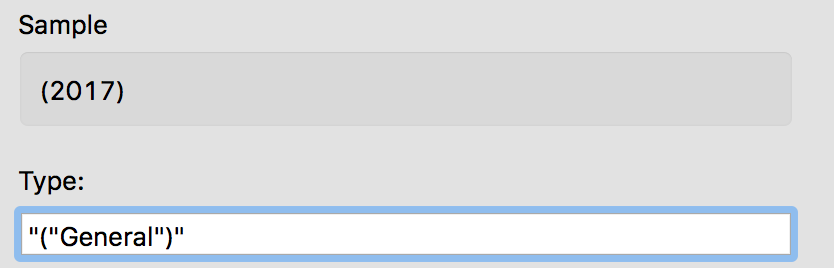If the value in cell is Text, you can enter “(“@”)” in type instead.

Step 5: Click OK to get result. All cells are enclosed with brackets properly.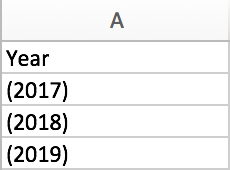Add Brackets for Cells by VBA Code in Excel

Step1: open your excel workbook and then click on “Visual Basic” command under DEVELOPER Tab, or just press “ALT+F11” shortcut.Step2: then the “Visual Basic Editor” window will appear.

Step3: click “Insert” ->”Module” to create a new module.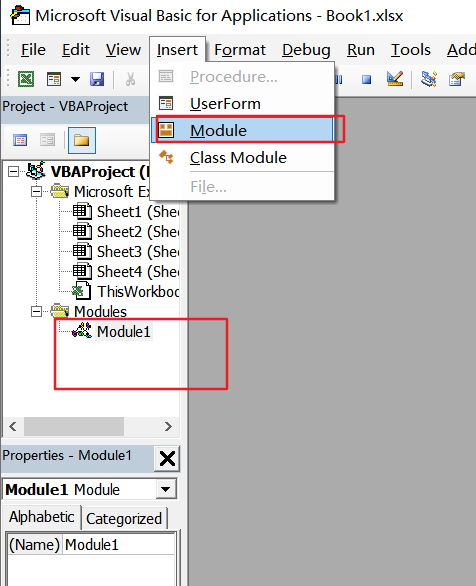Step4: paste the below VBA code  into the code window. Then clicking “Save” button.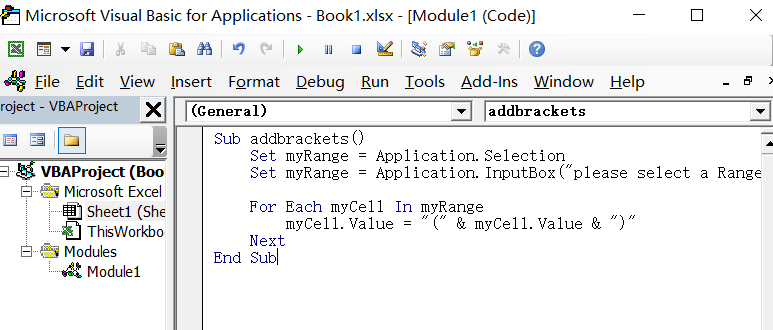Set myRange = Application.Selection

For Each myCell In myRange
myCell.Value = "(" & myCell.Value & ")"
Next
End Sub

Step5: back to the current worksheet, click on Macros button under Code group. then click Run button.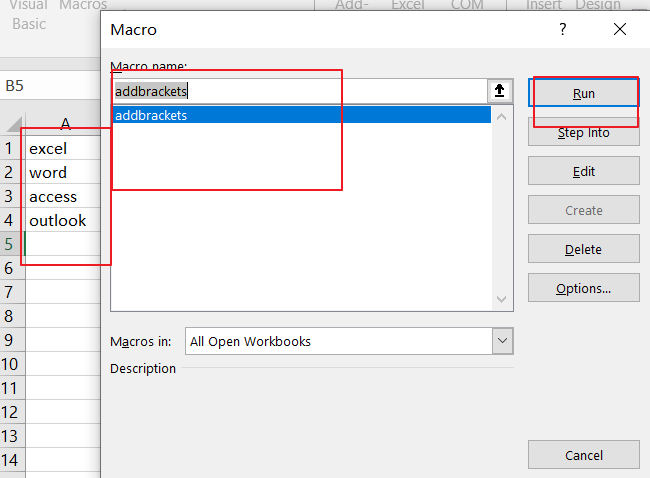Step6: you need to type one password to protect your current worksheet, click on Ok button.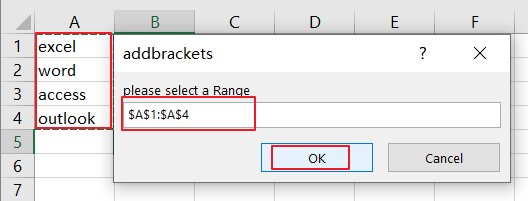Step7: you would see that the selected cells have been inserted brackets around text.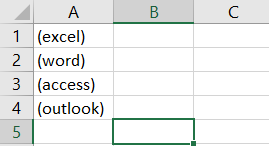Related Posts

VBA Macro For VLOOKUP From Another Sheet

In the previous post, you should know that how to fix or remove the #N/A error when using VLOOKUP formula to lookup value from another sheet. And this post will show you how to use VBA code to vlookup data ...

How To Insert Comments in Protected Worksheet in Excel

This post will show you how to allow comments in a protected worksheet in Excel. You can easily to insert comments into cells in a normal worksheet in Excel, but if want to insert a comment in a worksheet that ...

How To Convert Text to Upper Cases(Using VBA) in Excel

This post will show you how to switch from lower case to upper case in Excel. and I am going to show you two different ways of converting text to upper cases using formula or VBA macro in Excel 2013,Excel ...

How To Hide Every Other Row in Excel (Using VBA)

This post will show you how to hide alternate rows or columns in Excel or how to hide every third, fourth, fifth row or column in Excel. If you want to hide every other row in your current worksheet, how ...

How to Disable the Save As Prompt in Excel

This post will show you how to use a VBA Macro to save an Excel file and overwrite any existing file without a prompt so that you are going to get the little window that says file already exists do ...

How to Count Cells that Contain even or odd numbers in Excel

This post will guide you how to count the number of cells that contain odd or even numbers within a range of cells using a formula in Excel 2013/2016.How do I count cells that contain odd numbers through the use ...

How to Count Cells that Contain negative Numbers in Excel

This post will guide you how to count the number of cells that contain negative numbers within a range of cells using a formula in Excel 2013/2016.You can count the number of negative numbers in your data using easy functions ...

How to Count Cells Are Not Blank or Empty in Excel

This post will guide you how to count cells that are not blank or empty in a given range cells using a formula in Excel 2013/2016.How do I count the number of cells that are not blank in a particular ...

How to Count Cells Less Than a Specific Value in Excel

This post will guide you how to count the number of cells less than a particular numeric value in a given range cells using a formula in Excel 2013/2016. How do I count cells that are less than a specific ...

How to Count Cells Greater Than a Specific Value in Excel

This post will guide you how to count the number of cells greater than a particular numeric value in a given range cells using a formula in Excel 2013/2016. How do I count cells that are greater than a specific ...

Sidebar